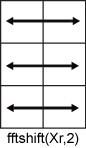# fftshift

## 语法

``Y = fftshift(X)``
``Y = fftshift(X,dim)``

## 说明

``Y = fftshift(X)` 通过将零频分量移动到数组中心，重新排列傅里叶变换 `X`。如果 `X` 是向量，则 `fftshift` 会将 `X` 的左右两半部分进行交换。如果 `X` 是矩阵，则 `fftshift` 会将 `X` 的第一象限与第三象限交换，将第二象限与第四象限交换。如果 `X` 是多维数组，则 `fftshift` 会沿每个维度交换 `X` 的半空间。`

``Y = fftshift(X,dim)` 沿 `X` 的维度 `dim` 执行运算。例如，如果 `X` 是矩阵，其行表示多个一维变换，则 `fftshift(X,2)` 会将 `X` 的每一行的左右两半部分进行交换。`

## 示例

```Xeven = [1 2 3 4 5 6]; fftshift(Xeven)```
```ans = 1×6 4 5 6 1 2 3 ```
```Xodd = [1 2 3 4 5 6 7]; fftshift(Xodd)```
```ans = 1×7 5 6 7 1 2 3 4 ```

```fs = 100; % sampling frequency t = 0:(1/fs):(10-1/fs); % time vector S = cos(2*pi*15*t); n = length(S); X = fft(S); f = (0:n-1)*(fs/n); %frequency range power = abs(X).^2/n; %power plot(f,power)```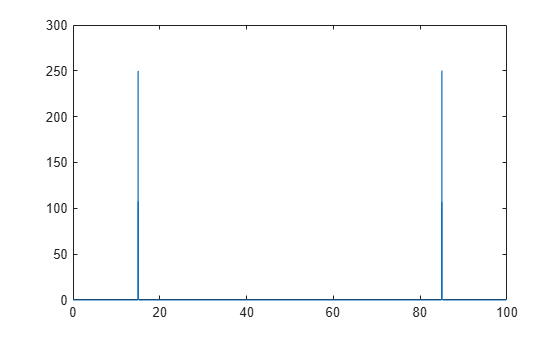```Y = fftshift(X); fshift = (-n/2:n/2-1)*(fs/n); % zero-centered frequency range powershift = abs(Y).^2/n; % zero-centered power plot(fshift,powershift)```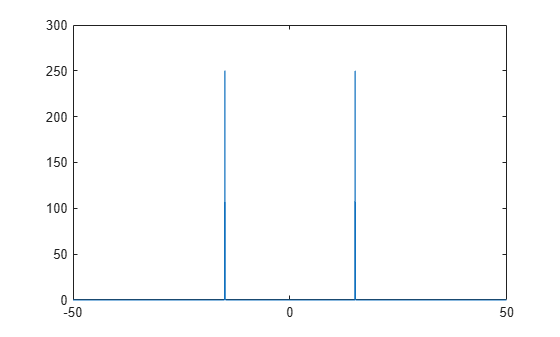```fs = 100; % sampling frequency t = 0:(1/fs):(10-1/fs); % time vector S1 = cos(2*pi*15*t); S2 = cos(2*pi*30*t); n = length(S1); A = [S1; S2]; X = fft(A,[],2); f = (0:n-1)*(fs/n); % frequency range power = abs(X).^2/n; % power plot(f,power(1,:),f,power(2,:))```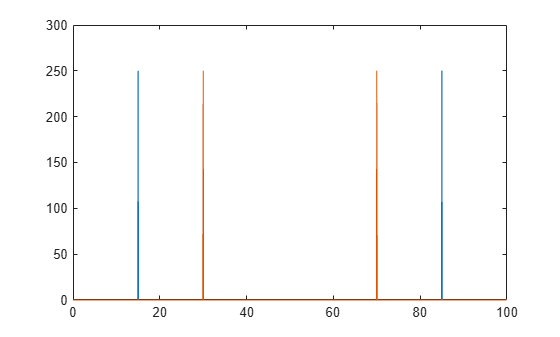```Y = fftshift(X,2); fshift = (-n/2:n/2-1)*(fs/n); % zero-centered frequency range powershift = abs(Y).^2/n; % zero-centered power plot(fshift,powershift(1,:),fshift,powershift(2,:))```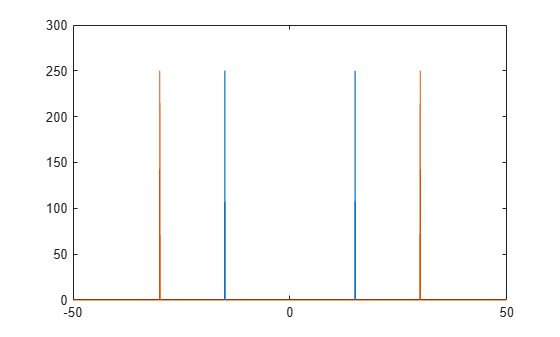## 输入参数

• 以输入矩阵 `Xc` 为例。运算 `fftshift(Xc,1)` 会将 `Xc` 的每一列的上下两半部分进行交换。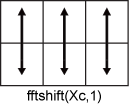• 假设有一个矩阵 `Xr`。运算 `fftshift(Xr,2)` 会将 `Xr` 的每一行的左右两半部分进行交换。